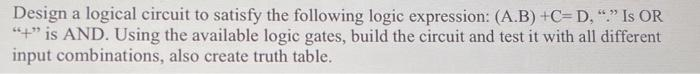Home / Expert Answers / Electrical Engineering / design-a-logical-circuit-to-satisfy-the-following-logic-expression-a-b-c-d-34-34-is-or-34-34-is-an-pa345

# (Solved): Design a logical circuit to satisfy the following logic expression: (A.B) +C=D, "." Is OR "+" is AN ...Design a logical circuit to satisfy the following logic expression: (A.B) , "." Is OR "+" is AND. Using the available logic gates, build the circuit and test it with all different input combinations, also create truth table.

We have an Answer from Expert